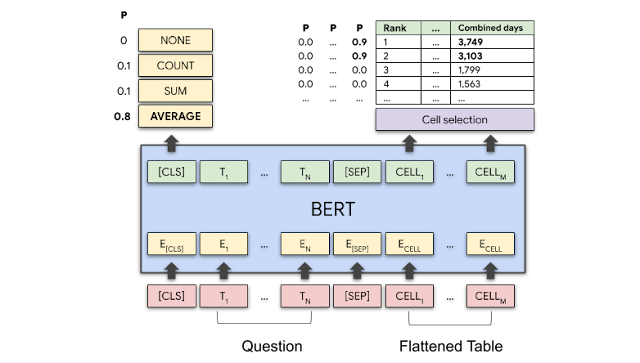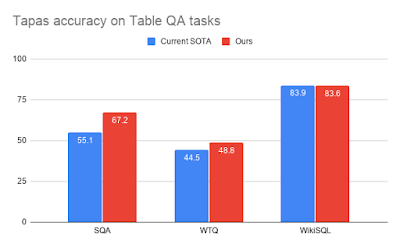# Using Neural Networks to Find Answers in Tables

Much of the world’s information is stored in the form of tables, which can be found on the web or in databases and documents. These might include anything from technical specifications of consumer products to financial and country development statistics, sports results and much more. Currently, one needs to manually look at these tables to find the answer to a question or rely on a service that gives answers to specific questions (e.g., about sports results). This information would be much more accessible and useful if it could be queried through natural language.

For example, the following figure shows a table with a number of questions that people might want to ask. The answer to these questions might be found in one, or multiple, cells in a table (“Which wrestler had the most number of reigns?”), or might require aggregating multiple table cells (“How many world champions are there with only one reign?”).A table and questions with the expected answers. Answers can be selected (#1, #4) or computed (#2, #3).
Many recent approaches apply traditional semantic parsing to this problem, where a natural language question is translated to an SQL-like database query that executes against a database to provide the answers. For example, the question “How many world champions are there with only one reign?” would be mapped to a query such as “`select count(*) where column("No. of reigns") == 1;`” and then executed to produce the answer. This approach often requires substantial engineering in order to generate syntactically and semantically valid queries and is difficult to scale to arbitrary questions rather than questions about very specific tables (such as sports results).

In, “TAPAS: Weakly Supervised Table Parsing via Pre-training”, accepted at ACL 2020, we take a different approach that extends the BERT architecture to encode the question jointly along with tabular data structure, resulting in a model that can then point directly to the answer. Instead of creating a model that works only for a single style of table, this approach results in a model that can be applied to tables from a wide range of domains. After pre-training on millions of Wikipedia tables, we show that our approach exhibits competitive accuracy on three academic table question-answering (QA) datasets. Additionally, in order to facilitate more exciting research in this area, we have open-sourced the code for training and testing the models as well as the models pre-trained on Wikipedia tables, available at our GitHub repo.

How to Process a Question
To process a question such as “Average time as champion for top 2 wrestlers?”, our model jointly encodes the question as well as the table content row by row using a BERT model that is extended with special embeddings to encode the table structure.

The key addition to the transformer-based BERT model are the extra embeddings that are used to encode the structured input. We rely on learned embeddings for the column index, the row index and one special rank index, which indicates the order of elements in numerical columns. The following image shows how all of these are added together at the input and fed into the transformer layers. The figure below illustrates how the question is encoded, together with the small table shown on the left. Each cell token has a special embedding that indicates its row, column and numeric rank within the column.BERT layer input: Every input token is represented as the sum of the embeddings of its word, absolute position, segment (whether it belongs to the question or table), column and row and numeric rank (the position the cell would have if the column was sorted by its numeric values).
The model has two outputs: 1) for each table cell, a score indicates the probability that this cell will be part of the answer and 2) an aggregation operation that indicates which operation (if any) is applied to produce the final answer. The following figure shows how, for the question “Average time as champion for top 2 wrestlers?”, the model should select the first two cells of the “Combined days” column and the “AVERAGE” operation with high probability.Model schematic: The BERT layer encodes both the question and table. The model outputs a probability for every aggregation operation and a selection probability for every table cell. For the question “Average time as champion for top 2 wrestlers?” the AVERAGE operation and the cells with the numbers 3,749 and 3,103 should have a high probability.
Pre-training
Using a method similar to how BERT is trained on text, we pre-trained our model on 6.2 million table-text pairs extracted from the English Wikipedia. During pre-training, the model learns to restore words in both table and text that have been replaced with a mask. We find that the model can do this with relatively high accuracy (71.4% of the masked tokens are restored correctly for tables unseen during training).Test answer accuracy for the weakly-supervised setup on three academic TableQA datasets.